# What does two pairs of equal sides mean at the end? What does "equal" mean?

• Module 2 Week 3 Day 10 Your Turn Part 1

What does two pairs of equal sides mean at the end? What does "equal" mean?

I still don't see how the shape is cyclic.

• Hi again!This question is tricky because it sort of makes you think that the quadrilateral is supposed to be cyclic inside the circle that is drawn in the diagram. That's what "cyclic quadrilateral" means, right? It's a quadrilateral with all of its vertices on the circle, inscribed inside the circle.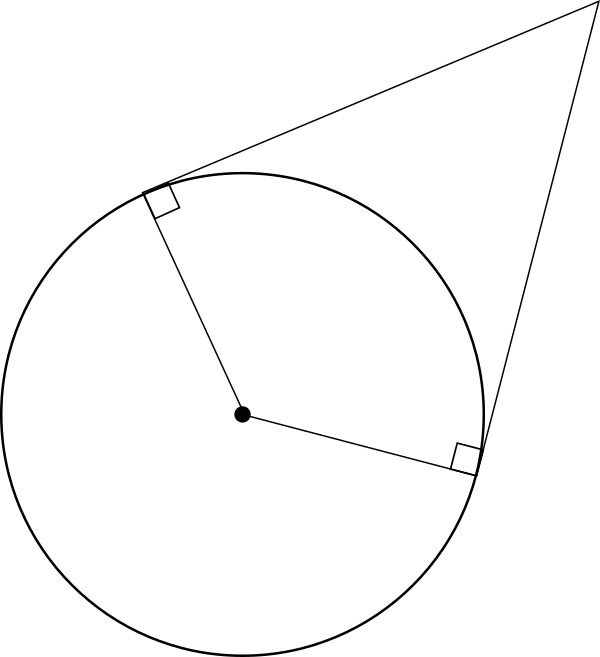But, actually, the sneaky circle in the diagram is a decoy... Well, at first it is useful, because we can use it to prove that the quadrilateral has two pairs of equal sides. (This means that of its four sides, two are the same shorter length, and the other two are also a same, but longer, length. Take a look at the two triangles below: they each are right triangles with a shorter leg that is a radius and hypotenuses which are the dotted line. Since these are right triangles, by the Pythagorean Theorem, their long legs (the tangent segments to the circle) must be the same length.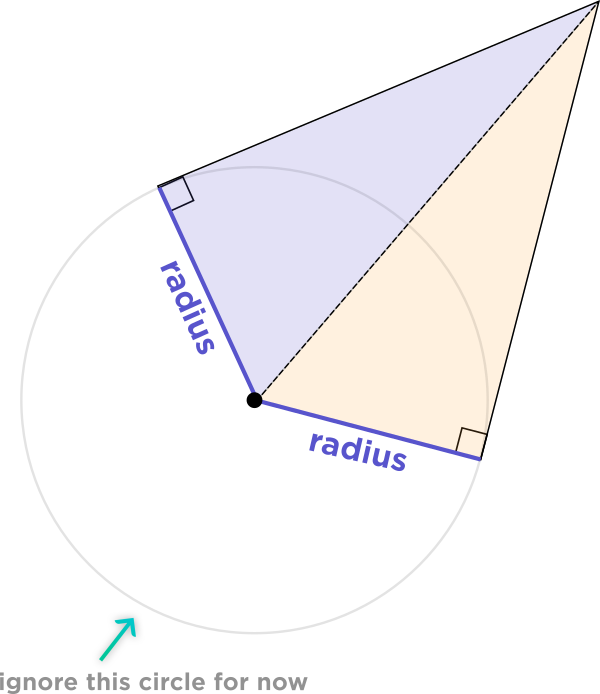This shows that the quadrilateral has two same length purple sides and two same length green sides.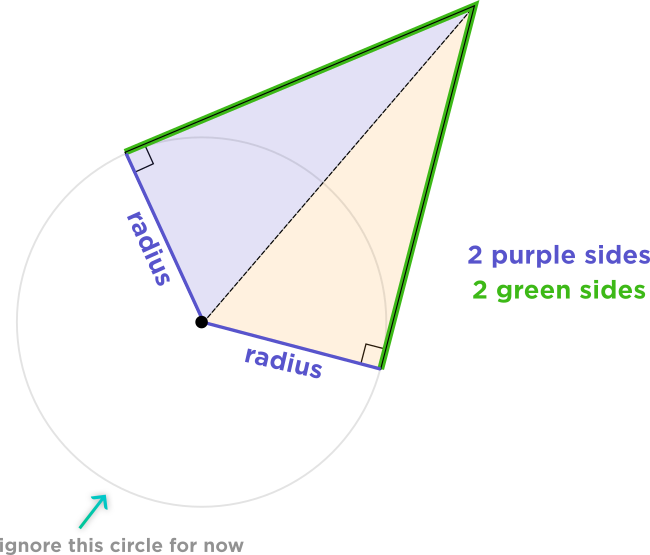Now, to show that it's cyclic, we have to ignore the grey circle for now. (I know it's there, but try to pretend it's not!) Instead, using the fact that we are given two opposite angles of \(140^{\circ}\) and \(40^{\circ},\) we know that these two angles add up to \(180^{\circ},\) which is half of a circle. They could both inscribe completely a circle, but a circle which is a different circle!

The yellow angle inscribes the yellow arc of the circle.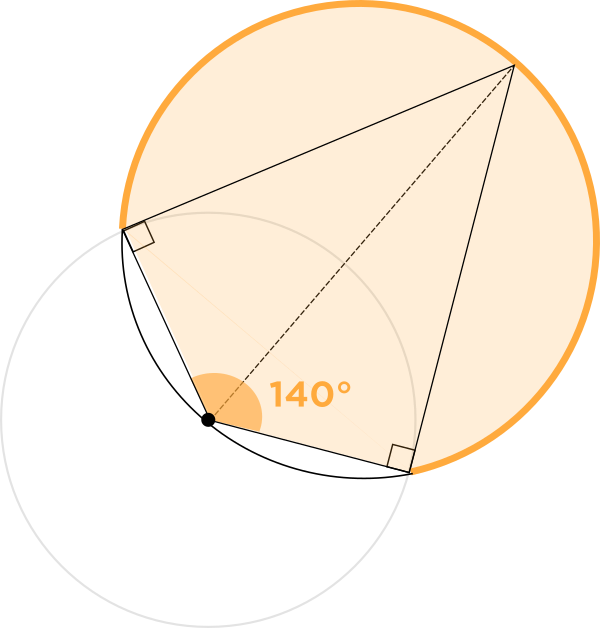And the green angle inscribes the green arc of the circle.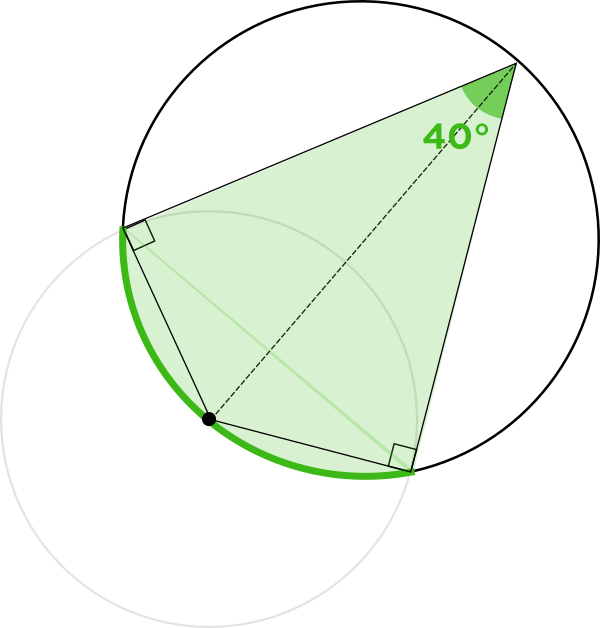And, as a check, the right angle inscribes an arc of the circle which have the endpoints of a diameter. This is correct, because an inscribed angle is half of its inscribed arc, so a \(90^{\circ}\) angle inscribes a \(180^{\circ}\) semicircular arc.

Since we can draw a circle circumscribed around the quadrilateral (with the vertices on the circle), this is a cyclic quadrilateral!